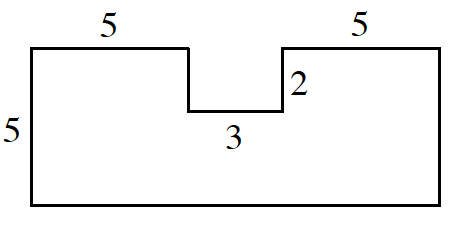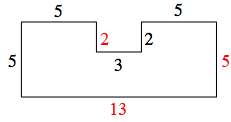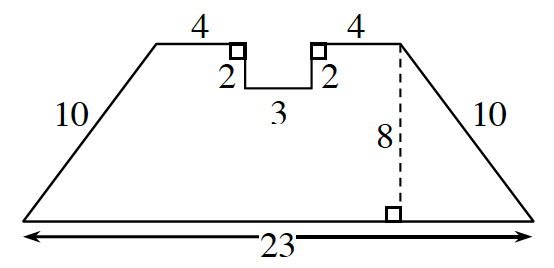### Home > CC2MN > Chapter 9 Unit 9 > Lesson CC2: 9.2.1 > Problem9-60

9-60.

Find the area and perimeter of each shape below. Show your steps and work.  Homework Help ✎

1. All angles are right angles.Label the missing sides.One method for calculating the area is to think of the shape as a large rectangle with a small ($2\times3$) rectangle missing.

$\text{Perimeter}=40$ units
$\text{Area}=65-6=59$ units2

1.Area of a trapezoid.

$\text{Area}=\left(\frac{1}{2}\right)(\text{base}_1+\text{base}_2)(\text{height})$